# Binary Fractals

#### Introduction

This is an example of one of the fractals made through a recursion algorith which will be described below. These fractals can all be made more simply with Hyperbolic Iterated Function Systems using affine maps, but the algorithm described here is different.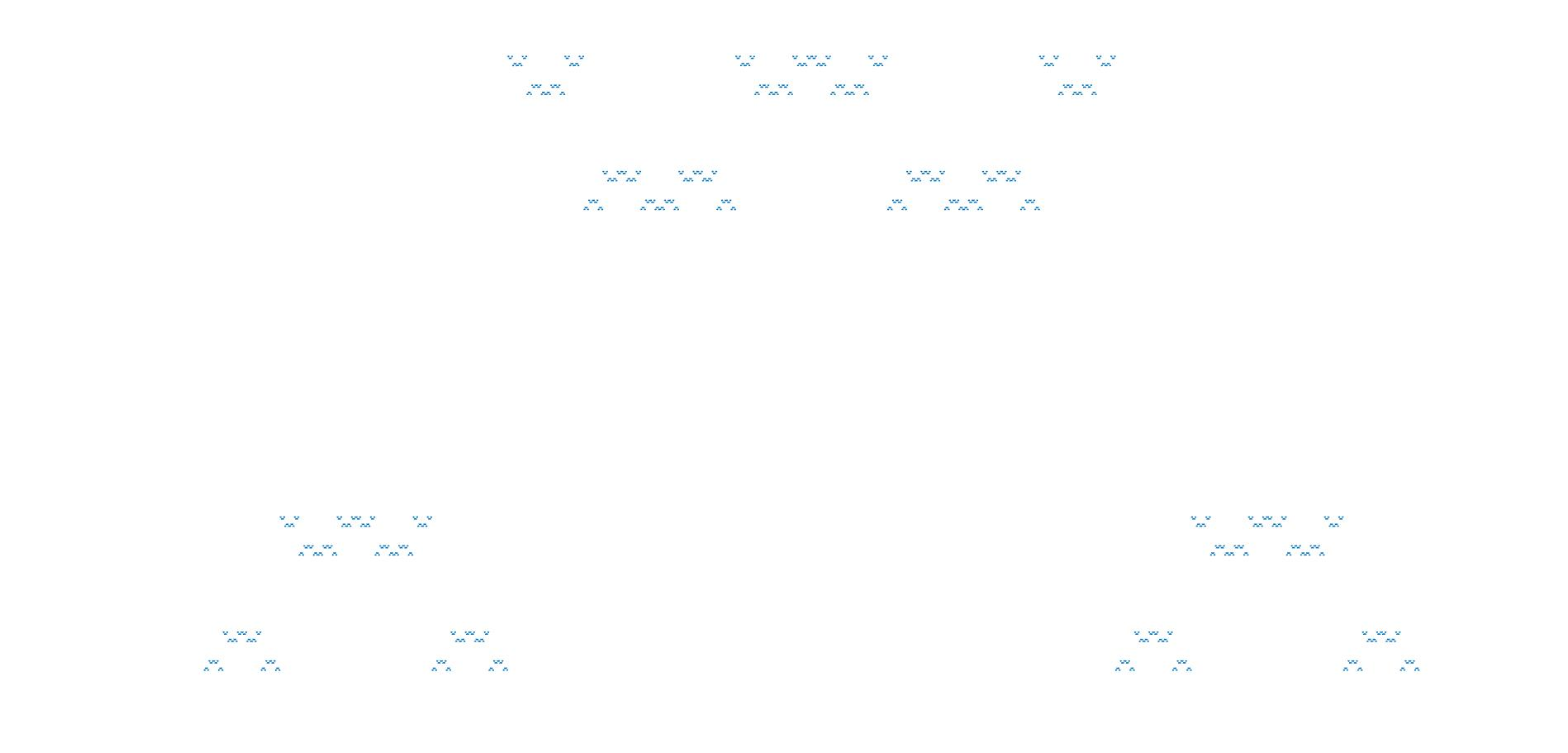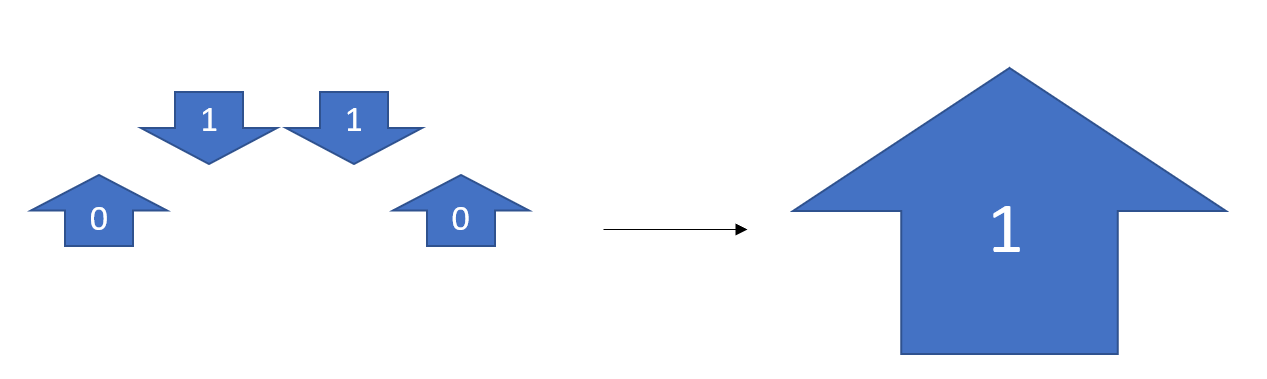Consider a binary code where each digit represents an arrow, zero=up and one=down. The first four would go up, down, down, up and imagine the "down" arrows would be above the "up" ones. If you squint really hard at these first four arrows, they kind of look like one big up arrow. Let's continue with that interpretation; make the next up, down, down, up, except each arrow is really four little ones, with the big down arrows being made from down, up, up, down. So 0 1 1 0 = 0110 1001 1001 0110. This process can go on forever; everytime you finish the four arrows, you just go up to the next size level and repeat.

As a brief aside, this binary sequence (0110 1001 1001 0110 1001 etc.) is called the Thue-Morse sequence and it can be attained by starting with zero and appending the opposite of the sequence to the end. For example, to 0 add 1, to 01 add 10, to 0110 add 1001, etc. This produces the exact same sequence.

#### Making the Fractals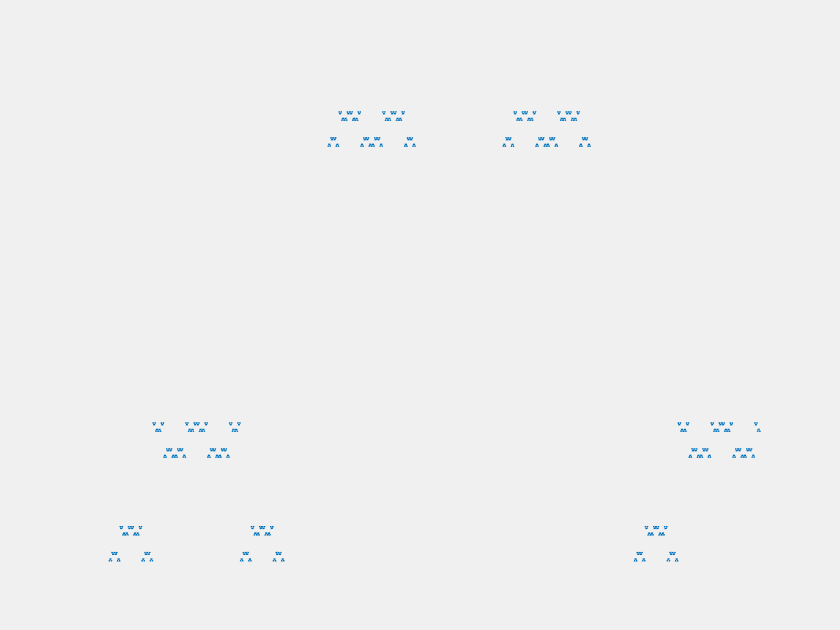When trying to write an algorithm that turns this sequence into fractals, a different approach is preferable. Let's work level by level, by levels I mean when we have $$4^n$$ points, ex: n=0 is 1, n=1 is 0110, n=2 is 0110 1001 1001 0110, etc. To move towards larger layers, we map each number in our input layer to a sequence on 4 numbers in the next layer. 1 maps to 0110 and 0 maps to 1001. We can also think of n as the number of times we have executed the expansion algorithm. To make the fractal from this sequence, we plot a point for every number in the sequence. The horizontal position of the points are determined by the index of thier number, i.e. the nth point is at x=n. The vertical positions change as we go, and when a number maps to the next layer, its daughter numbers inherit its vertical position. To make fractals, we must hve scale invariance, as this determines how the vertical positions will move. Upon computing the nth layer, we will move every '1' number's vertical position up by $$4^{-n}$$. This will preserve the scale invariance necessary to make the object a fractal. Do this process about 10 times, and the output plot is the one seen above, in the picture and gif.

It turns out that this algorithm can be generalized. We chose 0110 to be what 1 maps to, but we could have chosen anything. Let's call the binary sequence to which one maps: 'one map'. So for any given one map, zero will map to the inverse. We also need to change the scaling to keep the shape equivalent at all scales. For a one map that is m digits long, the vertical distance we move 1's up by each iteration is $$m^{n}$$, where n is the layer. MATLAB code that executes this algorithm can be found here. Here's some examples of the output...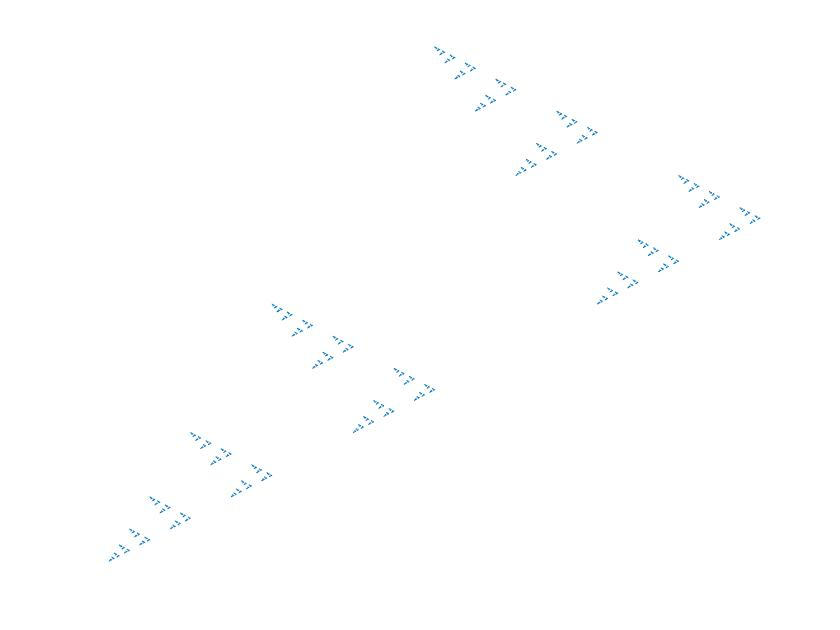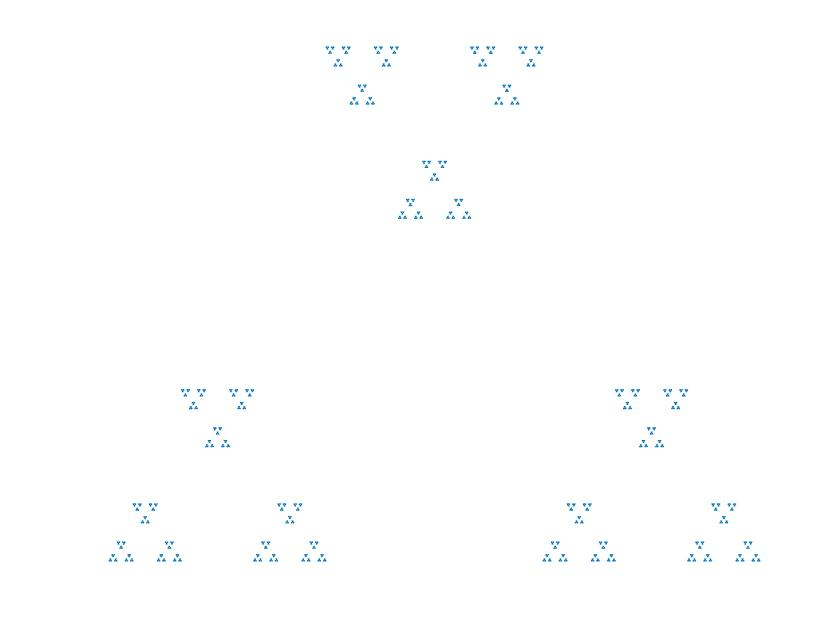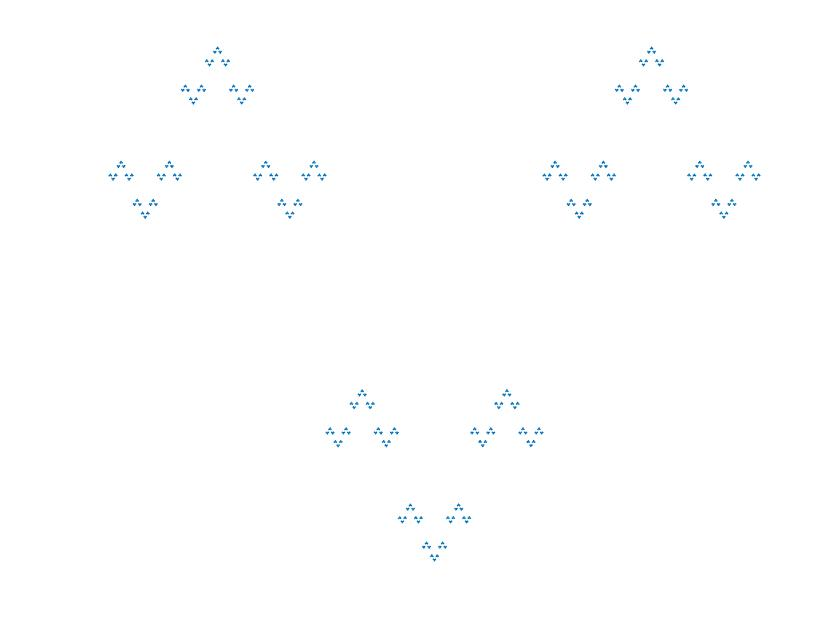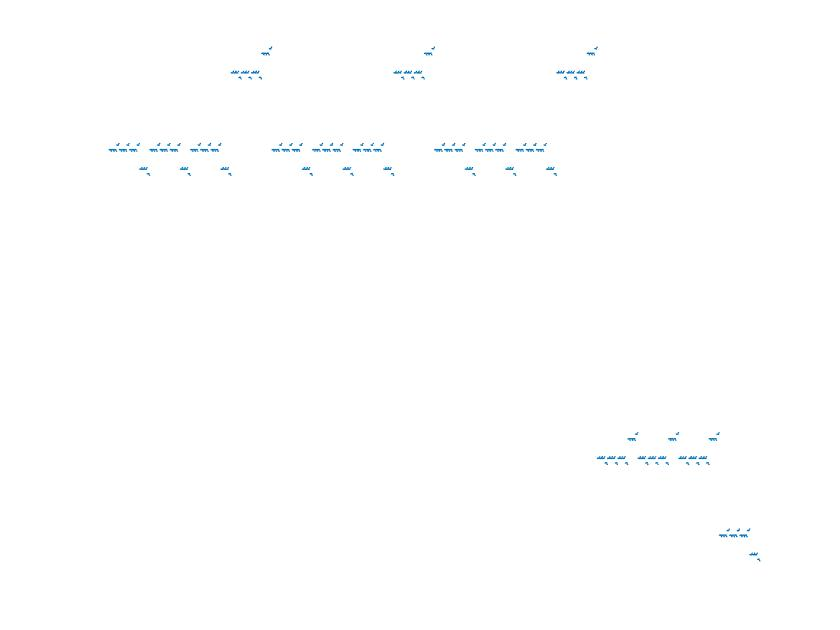Their one maps are: top left=01, top right=010, bottom left=101, bottom right=1110. As the one maps get longer, they all start to look quite similar. The top left (one map=01) fractal is actually featured in a paper. After staring at these fractals for awhile, you might notice that you can quickly tell what the one map is by simply looking at the fractal. Try to identify these two: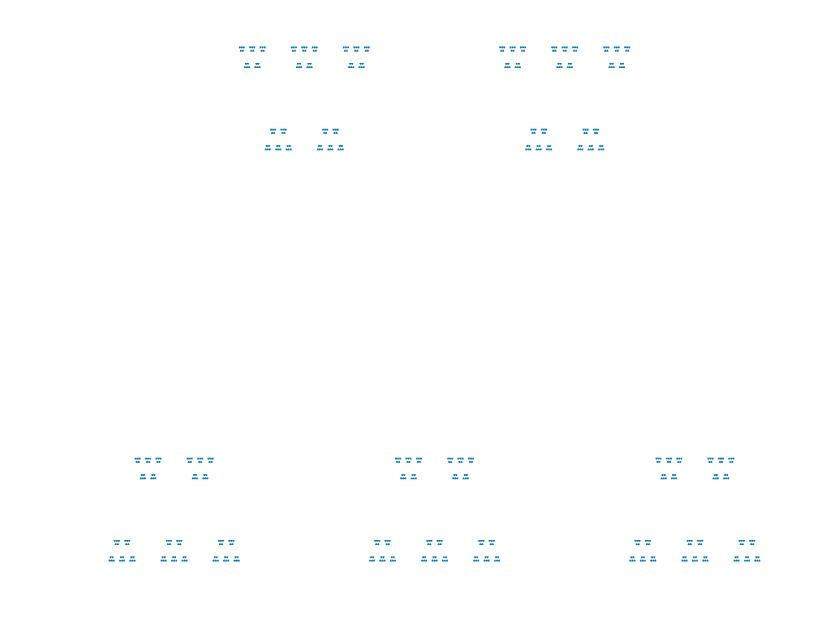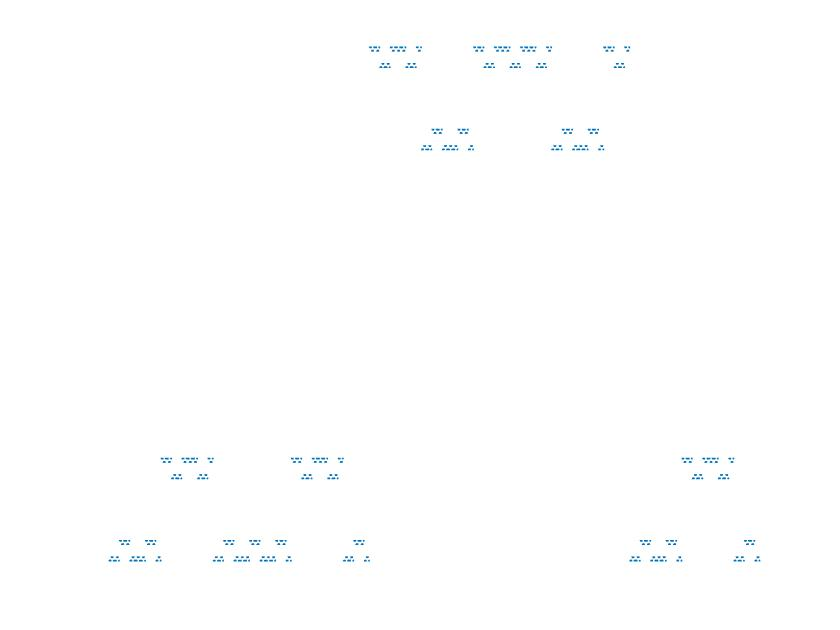Did you guess 01010 and 00110?

Here is one last zoom gif.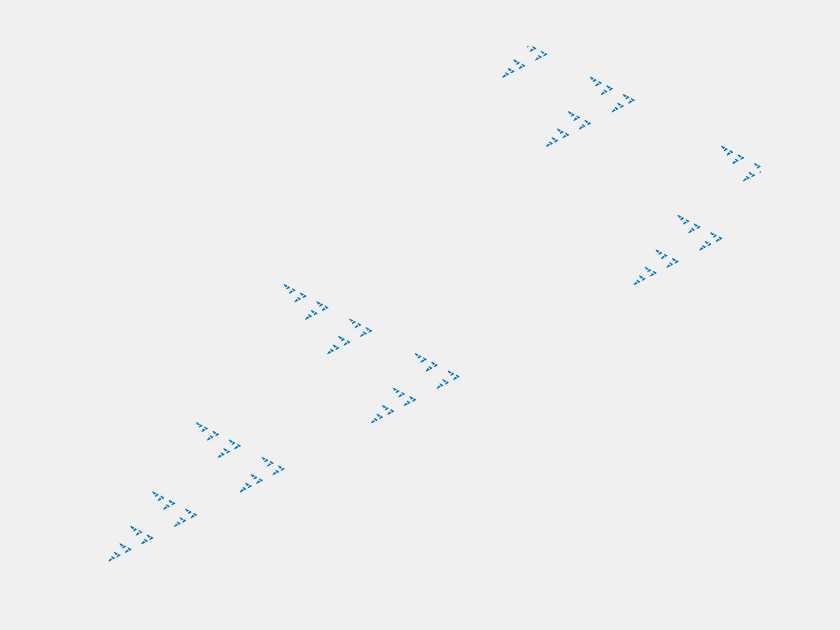Last Updated: 9/26/2020